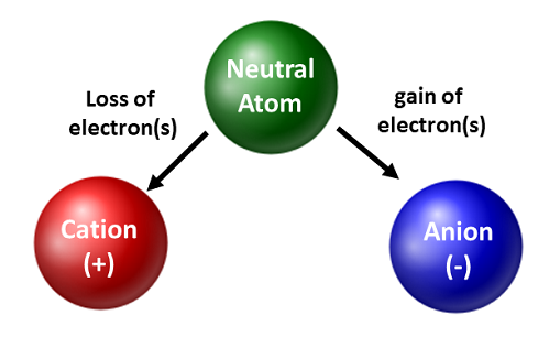# Cells and Ions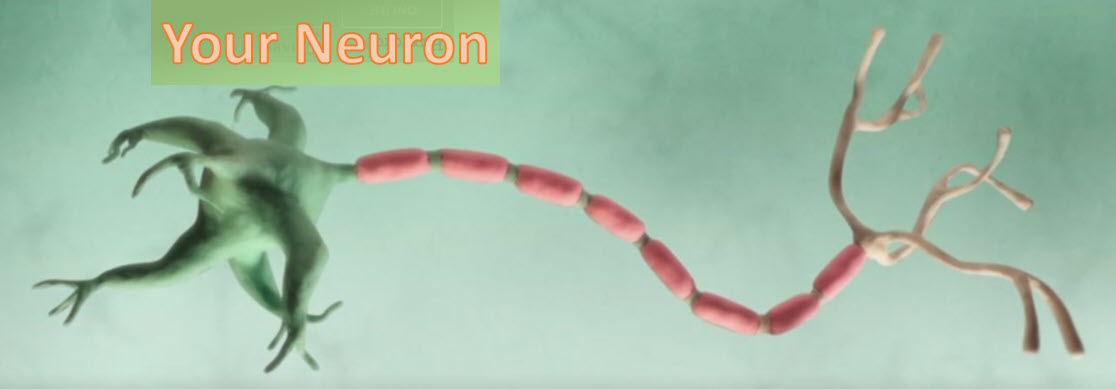This is a drawing of one of the 86 billion or so neuron cells that make up your brain. If your interested in understanding how these machines work follow along.

# 1.0 The Cell

The cell is the fundamental unit of organization of the brain and its key defining feature is the cell membrane. It separates the inside of a cell from the outside of a cell. When the cell membrane evolved, some three billion years ago, its primary advantage was to offer a stable intracellular environment for chemical reactions to take place that wouldn't be affected by changes in the extracellular environment. To do that evolution used lipids to create the cell membrane. This is how that works

The cell membrane is made of phospholipids with the chemical signature shown below. Each of these consists of phosphate containing head , and twin long hydrocarbon chains.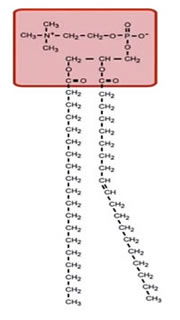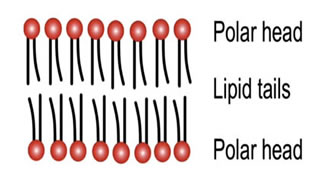The chains are lipophillc non-polar and therefor they are hydrophobic, they don't like to interact with water. The phosphate heads are highly polar and carry a strong negative charge. They like to interact with water that is they are hydrophilc.

## Lipid Bilayer

If you were to collect a few million of these of these lipids and drop them in water they amazingly, spontaneously form a planar lipid bilayer membrane.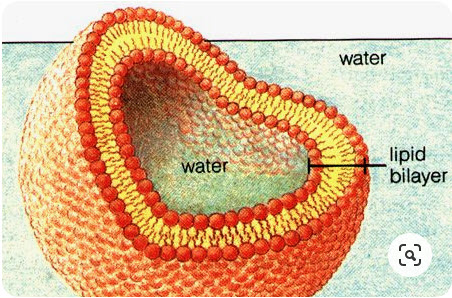The lipid membrane is:

• Higly permeable to gases and small uncharged molecules
• Limited permeability to water
• Impermeable to ions and charged molecules

## Ions

Ions are the most important element in the operation of the brain. These are charged, and it is this charge that forms the basis of electrical signaling in the nervous system. Because the membrane is impervious to ions means that there can be different concentrations of ions on the two sides. In a typical cell, there is a high concentration of positively-charged potassium ions on the inside, and on the outside (the extracellular solution) there is a high concentration of positively-charged sodium ions and a high concentration of negatively-charged chloride ions.

So the extracellular solution is made of Sodiumchloride or salt - simple kitchen salt.

The membrane is very thin, about five nanometers, and this allows ions on one side of the membrane to react to the charge of ions on the other. This causes the membrane to act like a capacitor, it's a dielectric, with strong fields across the lipid membrane. This called the membrane potential.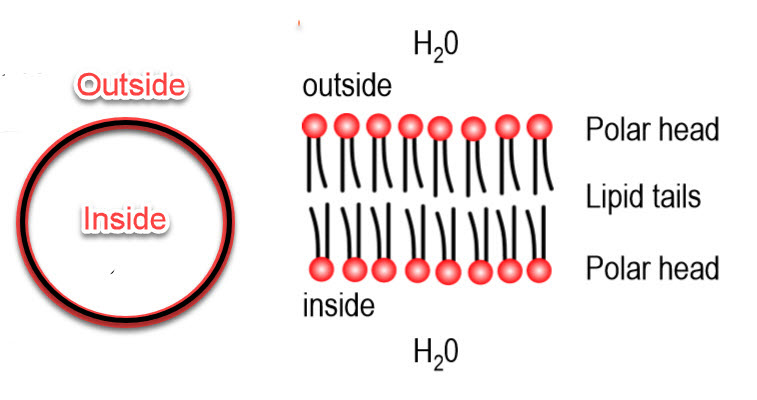## Movement of Charge

By measurement, the differential voltage between the inside and outside of the cell is about -100 mV. The outside, by convention, is always at 0 volts. The capacitance of the membrane is about one microfarad per square centimeter. Using these facts, we can determine how much positive change must move from the inside to the outside to give the inside that -100 mV change.

We know that the area of a small circular cell has a radius of around 10 micrometers, which puts the capacitance at about 10 picofarads. We also know that the voltage across a capacitor V is equal to the charge of the capacitor Q in coulombs divided by the capacitance C.

Where:

• C the capacitance of the cell ( 10 X 10-12 farad) and
• Q charge in coulombs
• V the voltage across the cell (0.1 Volt)

therefor:

Q = 1 X 10 -12 coulomb or 1 picocoulomb (pC)

The inside of the membrane contains a large number of positively charged potassium ions. How many of these would be required to move to make that 100 mVolt of charge.

Each ion carries a single charge and that charge is given by the elementary charge of an electron or 1.6 X 10 -19 coulomb:

Therefor 1 X 10-12 Coulomb divided by 1.6 X 10 -19 ~ six million ions that need to move from the inside to the outside to produce 100 mV differential.

That seems like a large number but there are about 1 X 10 11 potassium ions inside a typical cell so only a small number of ions available need to be moved to make this large change.

So how do these ions move across the membrane? The answer is 'ION CHANNELS'

## Ion Channels

There are several types of Ion channels. The ones we are dealing with here are the 'Always open' and 'Voltage-gated'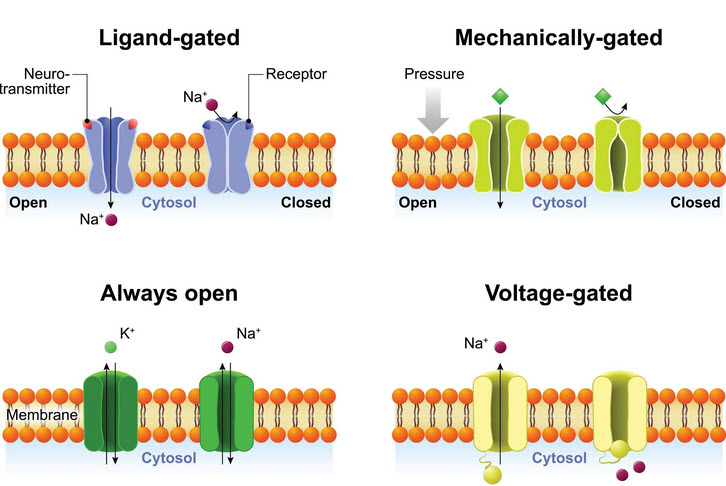The phospholipid bilayer isn't itself permeable to ions. So, how do ions move across the cell membrane? How does the membrane potential change? This is done by ion channels that traverse the membrane. They have pores in the middle that allows Potassium Sodium and Chloride ions to move through

This video depicts the operation of ion channels as they move Potassium ions and their positive charge from one side of the membrane to the other.

The video shows the operation of only Potassium channels but other channels exist as well:

• Potassium - K+
• Sodium - Na+ and
• Chloride - Ci-

The operation of the complete set of channels is complex and but well understood. Thankfully we do not have to have a complete understanding of these molecules to move forward.

## Membrane Potential

We know that the membrane acts as a capacitor and that ion channels act as conductors for specific ions. We can now work to see how the cell properties of electrochemical diffusion determine the membrane potential. Consider a single piece of the membrane with a potassium-selective ion channel as shown below. There are two main forces at work, the concentration gradient and the electrical gradient

For example if the potassium concentration is high inside the cell compared to the outside then the potassium will flow down the gradient to the outside. Since there is a large amount of potassium inside (shown by the large K+) as compared with the outside a concentration gradient exists that allows diffusion across the membrane.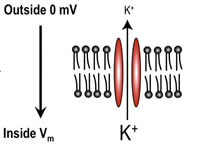### Electrical Force

There is a force applied to any charged ion that depends upon the spatial gradient of the electric field. This is one of the major determines of ionic movement and is show by the equation below.

$F = − q d V d X$

If we have a negative potential on the inside then potassium ions will be attracted to the inside down the electric-field gradient.

The concentration gradient and the electrical gradient oppose each other and precisely balance each other out at one specific potential - about -90 mV. This 'reversal potential this the point at which there's no net flux of ions.

The Nernst equilibrium equation for potassium ions is used to calculate this value.

$E k + = R T z F ln [ K + ] o [ K + ] i$

Where:

• Ek is the reversal potential for potassium
• Ko outside = 5 mM
• Ki inside = 150 mM
• R is Avogadro's gas constant
• T is Temperature in kelvins
• Z is the charge on the ion (for Potassium +1, calcium +2, chloride -1)
• F is the electrical force

RT over zF multiplies the logarithm of the ratio of the outside ionic concentration to the inside. This produces ~-90 mV reversal potential for Potassium.

Although potassium is a very important ion it is not the only one. Plugging values for different concentrations provide the equilibrium potential for some of the others..

Equilibrium Potential

Ion Inside Outside Name E ion
K+ 150 mm 4 mM Potassium -97 mV
Na+ 12 mM 145 mM Sodium +67 mV
Ci- 5 mM 120 mM Chloride -85 mV
Ca2+ 100 nM 1 mM Calcium +123 MV

Since each cell has a mixture of potassium, sodium and chloride that effect the membrane potential the result is a equilibrium potential of about -76 mV. This is called the resting potential - VRest.

## Next Cell Time Constant

An atom or molecule with a net electric charge due to the loss or gain of one or more electrons.
"Potassium ions"